CLAT  >  Test: Quantitative Techniques- 5

# Test: Quantitative Techniques- 5

Test Description

## 15 Questions MCQ Test Mock Test Series for CLAT | Test: Quantitative Techniques- 5

Test: Quantitative Techniques- 5 for CLAT 2023 is part of Mock Test Series for CLAT preparation. The Test: Quantitative Techniques- 5 questions and answers have been prepared according to the CLAT exam syllabus.The Test: Quantitative Techniques- 5 MCQs are made for CLAT 2023 Exam. Find important definitions, questions, notes, meanings, examples, exercises, MCQs and online tests for Test: Quantitative Techniques- 5 below.
Solutions of Test: Quantitative Techniques- 5 questions in English are available as part of our Mock Test Series for CLAT for CLAT & Test: Quantitative Techniques- 5 solutions in Hindi for Mock Test Series for CLAT course. Download more important topics, notes, lectures and mock test series for CLAT Exam by signing up for free. Attempt Test: Quantitative Techniques- 5 | 15 questions in 12 minutes | Mock test for CLAT preparation | Free important questions MCQ to study Mock Test Series for CLAT for CLAT Exam | Download free PDF with solutions
 1 Crore+ students have signed up on EduRev. Have you?
Test: Quantitative Techniques- 5 - Question 1

### Directions: Study the following graph carefully to answer the questions –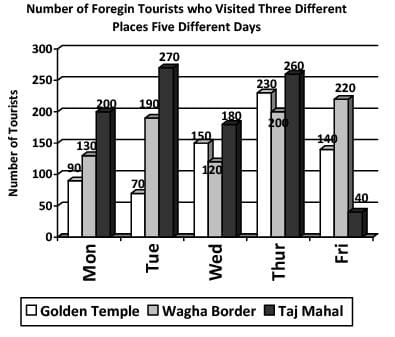Q. What is the average number of foreign tourists who visited Wagha Border on all the days together?

Detailed Solution for Test: Quantitative Techniques- 5 - Question 1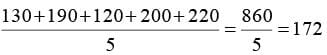Test: Quantitative Techniques- 5 - Question 2

### Directions: Study the following graph carefully to answer the questions –Q. On which day was the difference between the number of foreign tourists who visited Taj Mahal and Golden Temple, second highest?

Test: Quantitative Techniques- 5 - Question 3

### Directions: Study the following graph carefully to answer the questions –Q. What is the respective ratio between the number of foreign tourists who visited Taj Mahal on Thursday and the number of foreign tourists who visited Golden Temple on Friday?

Test: Quantitative Techniques- 5 - Question 4

Directions: Study the following graph carefully to answer the questions –Q. On which day was the total number of foreign tourists who visited all the three places together second lowest?

Test: Quantitative Techniques- 5 - Question 5

Directions: Study the following graph carefully to answer the questions –Q. Total number of foreign tourists who visited all the three places on Tuesday together is what per cent of the total number of foreign tourists who visited Wagha Border on Monday and Wednesday together?

Detailed Solution for Test: Quantitative Techniques- 5 - Question 5

The correct option is D.

Explanation-

Total no. of foreign tourists who visited all the three places on tuesday = 70+190+270 = 530

Total no. of foreign tourists who visited wagha border on monday and wednesday = 130+120 = 250

Percentage of  the total number of foreign tourists who visited Wagha Border on Monday and Wednesday together = 530/250*100 = 212.

Test: Quantitative Techniques- 5 - Question 6

Directions: Study the following piechart carefully and answer the questions given below:
Preferences of students for six chocolates in terms of degrees of angle in the pie-chart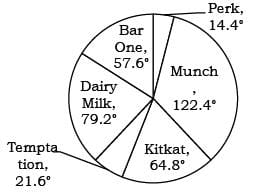Total number of students = 6800

Q. What is the difference between the total number of students who prefer Dairy Milk and Perk together and the total number of students who prefer Munch and Temptation together?

Detailed Solution for Test: Quantitative Techniques- 5 - Question 6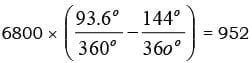Test: Quantitative Techniques- 5 - Question 7

Directions: Study the following piechart carefully and answer the questions given below:
Preferences of students for six chocolates in terms of degrees of angle in the pie-chartTotal number of students = 6800

Q. What is the ratio of the number of students who prefer Temptation to the number of students who prefer Dairy Milk?

Detailed Solution for Test: Quantitative Techniques- 5 - Question 7

21.6° : 79.2
3 : 11

Test: Quantitative Techniques- 5 - Question 8

Directions: Study the following piechart carefully and answer the questions given below:
Preferences of students for six chocolates in terms of degrees of angle in the pie-chartTotal number of students = 6800

Q. The number of students who prefer KitKat and Temptation together is what per cent of the total number of students?

Detailed Solution for Test: Quantitative Techniques- 5 - Question 8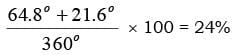Test: Quantitative Techniques- 5 - Question 9

Directions: Study the following piechart carefully and answer the questions given below:
Preferences of students for six chocolates in terms of degrees of angle in the pie-chartTotal number of students = 6800

Q. The number of students who prefer Perk is approximately what per cent of the number of students who prefer Munch?

Detailed Solution for Test: Quantitative Techniques- 5 - Question 9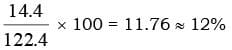Test: Quantitative Techniques- 5 - Question 10

Directions: Study the following piechart carefully and answer the questions given below:
Preferences of students for six chocolates in terms of degrees of angle in the pie-chartTotal number of students = 6800

Q. What is the ratio of the average of the students who prefer Dairy Milk, KitKat and Temptation together to those who prefer BarOne, Perk and Munch together?

Detailed Solution for Test: Quantitative Techniques- 5 - Question 10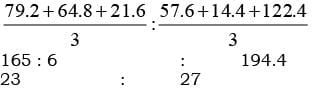Test: Quantitative Techniques- 5 - Question 11

Directions: Study the following table carefully. Some values are missing. Complete that based on given information in each question to answer the question.
The table shows the number of employees in an organization in 5 different cities with total employees being 2130 in the organization. Table also shows the percentage of employees working in 4 departments – HR, Finance, Software and Accounts with each employee in only 1 department.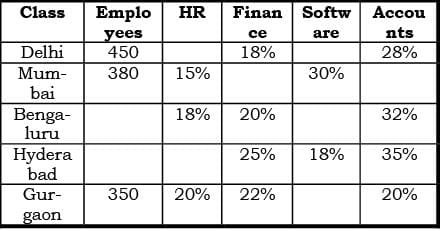Q. What is the total number of employees in Mumbai and Gurgaon who work in Software department?

Detailed Solution for Test: Quantitative Techniques- 5 - Question 11

In Gurgaon, Software % = 100 – (20 + 22 + 20) = 38%
Required Ans = (30/100) * 380 + (38/100)*350

Test: Quantitative Techniques- 5 - Question 12

Directions: Study the following table carefully. Some values are missing. Complete that based on given information in each question to answer the question.
The table shows the number of employees in an organization in 5 different cities with total employees being 2130 in the organization. Table also shows the percentage of employees working in 4 departments – HR, Finance, Software and Accounts with each employee in only 1 department.Q. If in Delhi, employees who work in Accounts department are 40% more than employees who work in HR department, then what is the number of employees who work in Software department in Delhi?

Detailed Solution for Test: Quantitative Techniques- 5 - Question 12

Let % of employees who work in HR in Delhi is x%.
So [28/100 * 450 – x/100 * 450]/[x/100 * 450] * 100 = 40
Which is [(28 - x)/x] * 100 = 40
Solve, x = 20
So % of employees who work in Software is 100 – (20 + 18 + 28) = 34%
So required ans = 34/100 * 450

Test: Quantitative Techniques- 5 - Question 13

Directions: Study the following table carefully. Some values are missing. Complete that based on given information in each question to answer the question.
The table shows the number of employees in an organization in 5 different cities with total employees being 2130 in the organization. Table also shows the percentage of employees working in 4 departments – HR, Finance, Software and Accounts with each employee in only 1 department.Q. If number of employees in Bengaluru is 10% less than number of employees in Hyderabad, then what is the difference between number of employees who work in Accounts department in these 2 cities and who work in Finance department in these 2 cities?

Detailed Solution for Test: Quantitative Techniques- 5 - Question 13

Total employees in Bengaluru and Hyderabad = 2130 – (450 + 380 + 350) = 950
So if in Hyderabad, employees is x, then in Bengaluru – 90/100 * x So x + 90x/100 = 950
Solve, x = 500,
so in Hyderabad = 500, and in Bengaluru = 90/100 * 500 = 450
So required answer = [32/100 * 450 + 35/100 * 500] – [20/100 * 450 + 25/100 * 500]
Or = 12/100 * 450 + 10/100 * 500

Test: Quantitative Techniques- 5 - Question 14

Directions: Study the following table carefully. Some values are missing. Complete that based on given information in each question to answer the question.
The table shows the number of employees in an organization in 5 different cities with total employees being 2130 in the organization. Table also shows the percentage of employees working in 4 departments – HR, Finance, Software and Accounts with each employee in only 1 department.Q. If a same criterion as taken in question 33 is taken, in which city the total employees who work in Finance and Accounts departments is more?

Detailed Solution for Test: Quantitative Techniques- 5 - Question 14

Delhi – (18 + 28)/100 * 450 = 207
Mumbai– 55/100 * 380 = 209
Bengaluru – (20 + 32)/100 * 450 = 234
Hyderabad – (25 + 35)/100 * 500 = 300
Gurgaon – (22 + 20)/100 * 350 = 147

Test: Quantitative Techniques- 5 - Question 15

Directions: Study the following table carefully. Some values are missing. Complete that based on given information in each question to answer the question.
The table shows the number of employees in an organization in 5 different cities with total employees being 2130 in the organization. Table also shows the percentage of employees working in 4 departments – HR, Finance, Software and Accounts with each employee in only 1 department.Q. If in Mumbai, number of employees who work in Accounts department is 19 more than the number of employees who work in Software department in Gurgaon, then what is the number of employees who work in Finance department in Mumbai?

Detailed Solution for Test: Quantitative Techniques- 5 - Question 15

% of employees who work in Software in Gurgaon = 100 – (20 + 22 + 20) = 38%
Number of employees who work in Software in Gurgaon = 38/100 * 350 = 133
So number of employees who work in Accounts in Mumbai is 133 + 19 = 152
So number of employees who work in Finance in Mumbai = 380 – [152 + ((15 + 30)/100 * 380)] = 57

## Mock Test Series for CLAT

2 videos|9 docs|77 tests
Information about Test: Quantitative Techniques- 5 Page
In this test you can find the Exam questions for Test: Quantitative Techniques- 5 solved & explained in the simplest way possible. Besides giving Questions and answers for Test: Quantitative Techniques- 5, EduRev gives you an ample number of Online tests for practice

## Mock Test Series for CLAT

2 videos|9 docs|77 tests(Scan QR code)